## Complex Fractions

If the numerator, denominator, or both contain fractions, then the expression is called a complex fraction. The fraction bar indicates division. To simplify a complex fraction:

1. Simplify both numerator and denominator expressions into single fraction expressions.

2. Divide the numerator by the denominator and simplify if possible.

##### Example 1

Simplify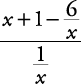.

Simplify numerator and denominator.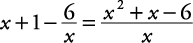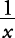is already a single fraction expression.Divide the numerator by the denominator and simplify.Therefore,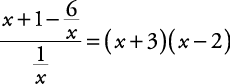##### Example 2

Simplify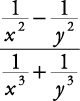.

Simplify numerator and denominator.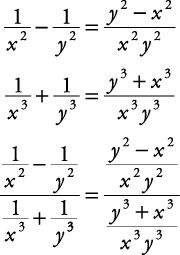Divide numerator by denominator and simplify.Therefore,Back to Top
A18ACD436D5A3997E3DA2573E3FD792A